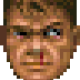• TreppenBananenHutstaender
•Topic Author
• Offline
6 years 9 months ago #1 by TreppenBananenHutstaender
Daltonize Shader was created by TreppenBananenHutstaender
Could someone port this Shader to ReShade Framework tried it myself but it didnt work:
```/*-----------------------------------------------------------.
/                     ColorBlind correction                   /
'-----------------------------------------------------------*/
// Daltonize (source http://www.daltonize.org/search/label/Daltonize)
float4 Daltonize( float4 input, float2 tex )
{
// RGB to LMS matrix conversion
float3 L = (17.8824f * input.r) + (43.5161f * input.g) + (4.11935f * input.b);
float3 M = (3.45565f * input.r) + (27.1554f * input.g) + (3.86714f * input.b);
float3 S = (0.0299566f * input.r) + (0.184309f * input.g) + (1.46709f * input.b);

// Simulate color blindness

#if ( Daltonize_type == 1) // Protanopia - reds are greatly reduced (1% men)
float l = 0.0f * L + 2.02344f * M + -2.52581f * S;
float m = 0.0f * L + 1.0f * M + 0.0f * S;
float s = 0.0f * L + 0.0f * M + 1.0f * S;
#endif

#if ( Daltonize_type == 2) // Deuteranopia - greens are greatly reduced (1% men)
float l = 1.0f * L + 0.0f * M + 0.0f * S;
float m = 0.494207f * L + 0.0f * M + 1.24827f * S;
float s = 0.0f * L + 0.0f * M + 1.0f * S;
#endif

#if ( Daltonize_type == 3) // Tritanopia - blues are greatly reduced (0.003% population)
float l = 1.0f * L + 0.0f * M + 0.0f * S;
float m = 0.0f * L + 1.0f * M + 0.0f * S;
float s = -0.395913f * L + 0.801109f * M + 0.0f * S;
#endif

// LMS to RGB matrix conversion
float4 error;
error.r = (0.0809444479f * l) + (-0.130504409f * m) + (0.116721066f * s);
error.g = (-0.0102485335f * l) + (0.0540193266f * m) + (-0.113614708f * s);
error.b = (-0.000365296938f * l) + (-0.00412161469f * m) + (0.693511405f * s);
error.a = 1;

// Isolate invisible colors to color vision deficiency (calculate error matrix)
error = (input - error);

// Shift colors towards visible spectrum (apply error modifications)
float4 correction;
correction.r = 0; // (error.r * 0.0) + (error.g * 0.0) + (error.b * 0.0);
correction.g = (error.r * 0.7) + (error.g * 1.0); // + (error.b * 0.0);
correction.b = (error.r * 0.7) + (error.b * 1.0); // + (error.g * 0.0);

// Add compensation to original values
correction = input + correction;
correction.a = input.a;

return correction.rgba;
}```

• Iddqd
•• Offline
6 years 9 months ago #2 by Iddqd
Replied by Iddqd on topic Daltonize Shader
But in mediator you cant enable i dont know why
You need enable effect in cfg manually
Installation: Copy lines and effect in arhive to you Framework

• TreppenBananenHutstaender
•Topic Author
• Offline
6 years 9 months ago #3 by TreppenBananenHutstaender
Replied by TreppenBananenHutstaender on topic Daltonize Shader
it's not working for me here is the log:
Warning: Spoiler!

•